15.1 Standing waves in wind instruments

 Page 1 / 1

Standing waves in wind instruments

A wind instrument is an instrument that is usually made with a pipe or thin tube. Examples of wind instruments are recorders, clarinets, flutes, organs etc.

When one plays a wind instrument, the air that is pushed through the pipe vibrates and standing waves are formed. Just like with strings, the wavelengths of the standing waveswill depend on the length of the pipe and whether it is open or closed at each end. Let's consider each of the following situations:

• A pipe with both ends open, like a flute or organ pipe.
• A pipe with one end open and one closed, like a clarinet.

If you blow across a small hole in a pipe or reed, it makes a sound. If both ends are open, standing waves will form according to [link] . You will notice that there is an anti-node at each end. In the next activity you will find how this affects the wavelengths.

Investigation : waves in a pipe open at both ends

This chart shows some standing waves in a pipe open at both ends. The pipe (shown with dashed lines) has length L.

1. Fill in the:
• number of nodes
• number of anti-nodes
• wavelength in terms of $L$
The first and last waves are done for you.
 Wave Nodes Antinodes Wavelength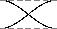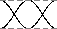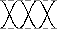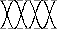2. Use the chart to find a formula for the wavelength in terms of the number of nodes.

The formula is different because there are more anti-nodes than nodes. The right formula is:

${\lambda }_{n}=\frac{2L}{n}$

Here, $n$ is still the number of nodes.

An open organ pipe is 0,853 m long. The speed of sound in air is 345 m.s ${}^{-1}$ . Can this pipe play middle C? (Middle C has a frequency of about 262 Hz)

The main frequency of a note is the fundamental frequency. The fundamental frequency of the open pipe has one node.

1. $f=\frac{v}{\lambda }$

We need to find the wavelength first.

$\begin{array}{ccc}\hfill \lambda & =& \frac{2L}{n}\hfill \\ & =& \frac{2\left(0,853\right)}{1}\hfill \\ & =& 1,706\phantom{\rule{4pt}{0ex}}\mathrm{m}\hfill \end{array}$
2. $\begin{array}{ccc}\hfill f& =& \frac{v}{\lambda }\hfill \\ & =& \frac{345}{1,706}\hfill \\ & =& 202\phantom{\rule{4pt}{0ex}}\mathrm{Hz}\hfill \end{array}$

This is lower than 262 Hz, so this pipe will not play middle C. We will need a shorter pipe for a higher pitch.

A flute can be modeled as a metal pipe open at both ends. (One end looks closed but the flute has an embouchure , or hole for the player to blow across. This hole is large enough for air to escape on that side as well.)If the fundamental note of a flute is middle C (262 Hz) , how long is the flute? The speed of sound in air is 345 m.s ${}^{-1}$ .

We can calculate the length of the flute from $\lambda =\frac{2L}{n}$ but:

1. $\begin{array}{ccc}\hfill f& =& \frac{v}{\lambda }\hfill \\ \hfill 262& =& \frac{345}{\lambda }\hfill \\ \hfill \lambda & =& \frac{345}{262}=1,32\phantom{\rule{4pt}{0ex}}\mathrm{m}\hfill \end{array}$
2. $\begin{array}{ccc}\hfill \lambda & =& \frac{2L}{n}\hfill \\ & =& \frac{2L}{1}\hfill \\ \hfill L& =& \frac{1,32}{2}=0,66\phantom{\rule{4pt}{0ex}}\mathrm{m}\hfill \end{array}$

Now let's look at a pipe that is open on one end and closed on the other. This pipe has a node at one end and an antinode at the other. An example of a musicalinstrument that has a node at one end and an antinode at the other is a clarinet. In the activity you will find out how the wavelengths are affected.

Investigation : waves in a pipe open at one end

This chart shows some standing waves in a pipe open at one end. The pipe (shown as dashed lines) has length L.

1. Fill in the:
• number of nodes
• number of anti-nodes
• wavelength in terms of $L$
The first and last waves are done for you.
 Wave Nodes Antinodes Wavelength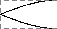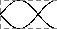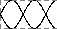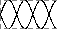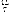2. Use the chart to find a formula for the wavelength in terms of the number of nodes.

The right formula for this pipe is:

${\lambda }_{n}=\frac{4L}{2n-1}$

A long wavelength has a low frequency and low pitch. If you took your pipe from the last example and covered one end, you should hear a much lower note! Also, the wavelengths of the harmonics for this tube are not integer multiples of each other.

A clarinet can be modeled as a wooden pipe closed on one end and open on the other. The player blows into a small slit on one end. A reed then vibrates in the mouthpiece. This makes the standing wave in the air. What is the fundamental frequency of a clarinet 60 cm long? The speed of sound in air is 345 m.s ${}^{-1}$ .

1. We are given:

$\begin{array}{ccc}\hfill L& =& 60\phantom{\rule{4pt}{0ex}}\mathrm{cm}\hfill \\ \hfill v& =& 345\phantom{\rule{4pt}{0ex}}\mathrm{m}.{\mathrm{s}}^{-1}\hfill \\ \hfill f& =& ?\hfill \end{array}$
2. $\begin{array}{ccc}\hfill \lambda & =& \frac{4L}{2n-1}\hfill \\ & =& \frac{4\left(0,60\right)}{2\left(1\right)-1}\hfill \\ & =& 2,4\phantom{\rule{4pt}{0ex}}\mathrm{m}\hfill \end{array}$
3. $\begin{array}{ccc}\hfill f& =& \frac{v}{\lambda }\hfill \\ & =& \frac{345}{2,4}\hfill \\ & =& 144\phantom{\rule{4pt}{0ex}}\mathrm{Hz}\hfill \end{array}$

This is closest to the D below middle C. This note is one of the lowest notes on a clarinet.

Musical scale

The 12 tone scale popular in Western music took centuries to develop. This scale is also called the 12-note Equal Tempered scale. It has an octave divided into 12 steps. (An octave is the main interval of most scales. If you double a frequency, you have raised the note one octave.) All steps have equal ratios of frequencies. But this scale is not perfect. If the octaves are in tune, all the other intervals are slightly mistuned. No interval is badly out of tune. But none is perfect.

For example, suppose the base note of a scale is a frequency of 110 Hz ( a low A). The first harmonic is 220 Hz. This note isalso an A, but is one octave higher. The second harmonic is at 330 Hz (close to an E). The third is 440 Hz (also an A). Butnot all the notes have such simple ratios. Middle C has a frequency of about 262 Hz. This is not a simple multiple of 110 Hz. So the interval between C and A is a little out of tune.

Many other types of tuning exist. Just Tempered scales are tuned so that all intervals are simple ratios of frequencies.There are also equal tempered scales with more or less notes per octave. Some scales use as many as 31 or 53 notes.

during a snooker competition ,a 200g ball A m moving with velocity va collide head on with a identical ball B that was at rest.A after the collision ball A remains at rest wile ball B moves on with a velocity of 4m/s? With what speed was ball a moving before the collision
a vector can be resolved into a horizontal component only
how to calculate normal force
how to calculate wavelength
Lizoh
Hello. How does a real gas behave under low temperature and high pressure?
does a vector quantity include force and distance?
yes
Itumeleng
what's the difference between a vector and a scalar?
Keaobaka
vector is the physical quantity with magnitude and direction Scalar is the Physical quantity with magnitude only
Bobo
Newton's second law of motion
Newton second law motin
Shife
Newton's second law of motion: When a resultant/net force acts on an object, the object will accelerate in the direction of the force at an acceleration directly proportional to the force and inversely proportional to the mass of the object.
Bobo
newtons third law of motion
Ayakha
what is tail to tail method
a person was standing in a stationary lift / elevator and scale shown is 490N and then 470N when the lift started to move. Did the lift go up or down?
down
Fabzeey
How do you state snell's law
The ratio of the sine of the angle of incidence in one medium to the sine of the angle of refraction in the other medium is constant
Boss
who was the first person to discover nuclears bomb
how to calculate resistance in grade 11
last year memo in 2018 June exam
Ngomane
can I have last june question paper2
Mike
yes
Ismael
hello
Amanda
hello
Patricia
is there any physics e-copy textbooks?
Patricia
states the Newton's second law of motion
In words it says:" when a net force is applied to an object of mass, It accelerates in the direction of the net force. The acceleration is directly proportional to the net force and inversely proportional to the mass".
Buhle
And in symbols: Fnet = ma
Buhle
thank you
Shallin
pleasure
Buhle
hello everyone
Ngomane
hello
Mzondi
hlw
manu
which degree is usually be a refractive angle before it complete a totally reflection?
Mzondi
90°
Boss
yes obviously 90 degree
manu
fnet=ma
Fabzeey
how does hydrogen bonds differ from London force
Hydrogen bonds are the strongest intermolecular forces and London forces are the weakest. Hydrogen bonds exist between polar molecules ( they are a special case of dipole-dipole forces ), while London forces occur between non-polar molecules.
Kagiso
how come the resultant force is 0
It's when you have equivalent forces going different directions then your resultant will be equal to zero
Temosho
describe what john's experiment proves about water molecules?By Edward Biton